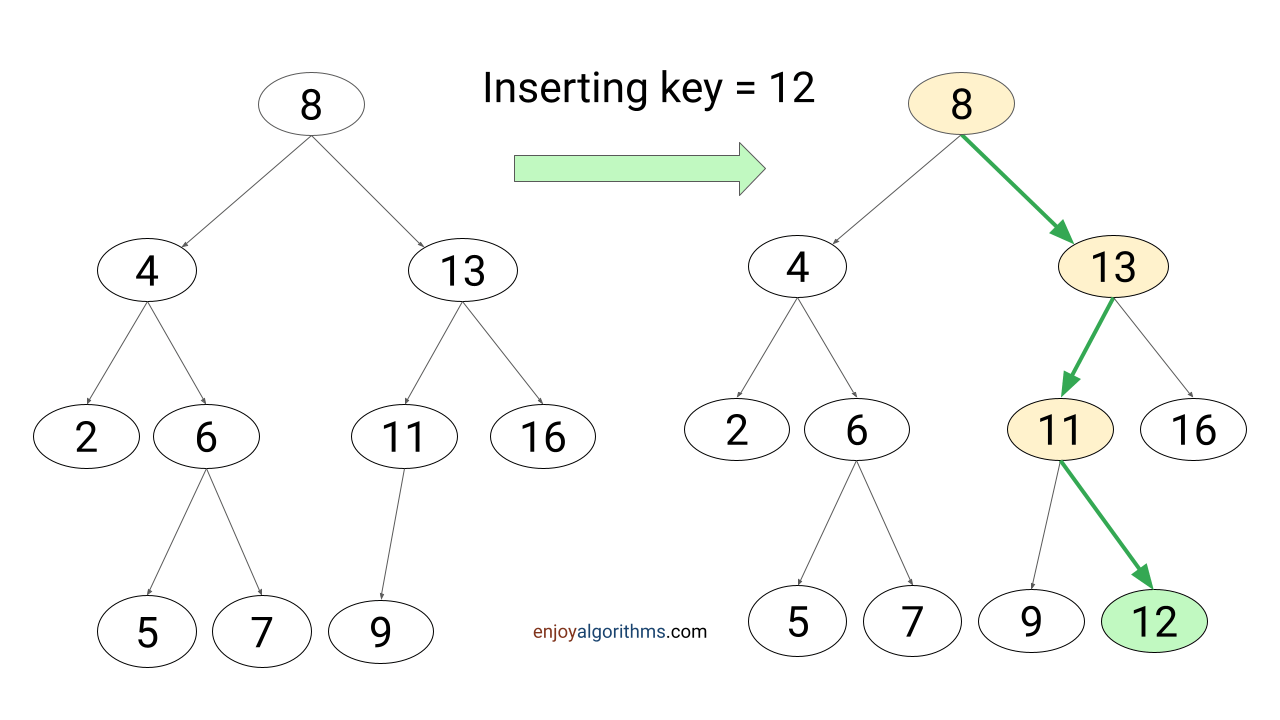# Insert Operation in Binary Search Tree (BST)

Problem statement: The root of the binary search tree and a key k is given. Write a program to insert key k into the binary search tree. As an output, we need to return the root of the modified BST. Note: BST structure will change after the insertion. So we need to perform insertion in such a way that the BST property continues to hold.

### Insertion in BST using recursion

The recursive implementation of the insert operation is similar to the recursive implementation of the search operation: If BST is empty, we return a new node containing key k as the root. Otherwise, if k is less than root->key, we recursively insert k into the left subtree. Similarly, if k is greater than root->key, we recursively insert k into the right subtree.

From another perspective, new key insertion always happens at one of the NULL links at the bottom of the tree. So based on a comparison with keys stored in the root-to-leaf path, recursion will find an appropriate NULL link to insert the new key. When it reaches the NULL link, we replace that NULL link with a new node containing key k.#### Solution pseudocode

``````BSTNode bstInsertRecursive(BSTNode root, int k)
{
if (root == NULL)
{
root = new BSTNode(k)
return root
}
else if (k < root->key)
root->left = bstInsertRecursive(root->left, k)

else if (k > root->key)
root->right = bstInsertRecursive(root->right, k)
return root
}``````

### Insertion in BST using iteration

We can simulate the above recursive solution iteratively using the while loop. The idea is simple: We need to traverse down the tree iteratively to find the appropriate NULL link to insert the new key.

For some node x in the path, if the x->key is greater than k, we follow the left pointer and go to the left subtree. Otherwise, we follow the right pointer and go to the right subtree. We continue this process in a while loop. By the end of the loop, we will be present at one of the NULL links, which occupies the position where we wish to place key k.

During the loop, we also need a trailing pointer as the parent of the current node because by the time we find the NULL link, the loop has proceeded one step beyond the node that needs to be changed. Think!

#### Solution steps

• We create a new BSTNode newnode with key k. If BST is empty, set newnode as the root and return it.
``````BSTNode newnode = new BSTNode(k)
if(root == NULL)
{
root = newnode
return root
}``````
• We define curr pointer and initialize it with root node.
• We define parent pointer and initialize it with NULL.
• After initialization, we run a while loop till curr != NULL. This loop causes these two pointers to move down the tree, going left or right depending on the comparison k with curr->key.
``````BSTNode curr = root
BSTNode parent = NULL
while (curr != NULL)
{
parent = curr
if (k < curr->key)
curr = curr->left
else
curr = curr->right
}``````
• By end of the loop, newnode containing key k will be child of parent pointer. So if k < parent->key, we assign newnode as the left child of parent. Otherwise, we assign newnode as the right child of parent.
``````if (k < parent->key)
parent->left = newnode
else
parent->right = newnode``````
• Finally, we return root node as an output.

#### Solution pseudocode

``````BSTNode bstInsertIterative(BSTNode root, int k)
{
BSTNode newnode = new BSTNode(k)
if(root == NULL)
{
root = newnode
return root
}
BSTNode curr = root
BSTNode parent = NULL
while (curr != NULL)
{
parent = curr
if (k < curr->key)
curr = curr->left
else
curr = curr->right
}
if (k < parent->key)
parent->left = newnode
else
parent->right = newnode

return root
}``````

### Time complexity analysis

At each step of recursion or iteration, we are performing O(1) operation and moving one level downward in the tree (till we reach the NULL link). So total number of comparison is equal to the height of BST. Time complexity of insert operation = The height of BST * O(1) = h * O(1) = O(h).

When BST is balanced, both left and right subtree will be of same size (n/2) and height of BST will be O(logn). So time complexity of insertion in a balanced BST = O(logn).

When BST is completely unbalanced, it will be a linear chain of nodes (one node at each level) and the height of such BST is O(n). So time complexity of insertion in a unbalanced BST = O(n).

### Space complexity analysis

We are using constant extra memory in iterative implementation, so space complexity of iterative insert operation in BST = O(1). In the recursive implementation, there will be one recursive call at each level of tree. So space complexity depends on the size of recursion call stack, which is equal to the height of the tree. So space complexity of recursive insert in BST = O(h)

• For balanced BST, space complexity = O(logn).
• For completely unbalanced BST, space complexity= O(n).

Enjoy learning, enjoy algorithms!

☆ 16-Week Live DSA Course
☆ 10-Week Live DSA Course